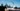# ES 提案：公有类字段

## 概述：

### 公有实例字段

``````const instFieldKey = Symbol('instFieldKey');
class MyClass {
instField = 1;
[instFieldKey] = 2; // computed key(计算字段键)
}
const instance = new MyClass();
assert.equal(instance.instField, 1);
assert.equal(instance[instFieldKey], 2);``````

### 公有静态字段

``````const staticFieldKey = Symbol('staticFieldKey');
class MyClass {
static staticField = 1;
static [staticFieldKey] = 2; // computed key(计算字段键)
}
assert.equal(MyClass.staticField, 1);
assert.equal(MyClass[staticFieldKey], 2);``````

## 公有实例字段与公有静态字段

### 公有实例字段

``````class MyClass {
constructor() {
this.counter = 0;
}
}
assert.equal(new MyClass().counter, 0);``````

``````class MyClass {
counter = 0;
constructor() {}
}
assert.equal(new MyClass().counter, 0);``````

``````class MyClass {
counter;
constructor() {}
}
assert.equal(new MyClass().counter, undefined);``````

### 公有静态字段

JavaScript 没有办法在类内部创建一个静态属性，你可以通过外部任务创建它：

``````class MyClass {}
MyClass.prop = 123;
assert.equal(MyClass.prop, 123);``````

``````class MyClass {
static get prop() {
return 123;
}
}
assert.equal(MyClass.prop, 123);``````

``````class MyClass {
static prop = 123;
}
assert.equal(MyClass.prop, 123);``````

## 举例：使用字段转换构造函数

``````class StringBuilder {
constructor() {
this.data = '';
}
this.data += str;
return this;
}
toString() {
return this.data;
}
}
assert.equal(
'Hello world!',
);``````

``````class StringBuilder {
data = '';
this.data += str;
return this;
}
toString() {
return this.data;
}
}``````

## 分配 vs 定义

### 分配属性

``````const proto = {
set prop(value) {
console.log('SETTER: ' + value);
},
};
const obj = {
__proto__: proto,
};
obj.prop = 123; // (A)assert.equal(obj.prop, undefined);

// Output:
// 'SETTER: 123'``````

``````class A {
set prop(value) {
console.log('SETTER: ' + value);
}
}
class B extends A {
constructor() {
super();
this.prop = 123; // (A)  }
}
assert.equal(new B().prop, undefined);

// Output:
// 'SETTER: 123'``````

## 定义属性

``````const proto = {
set prop(value) {
console.log('SETTER: ' + value);
},
};
const obj = {
__proto__: proto,
};
Object.defineProperty(obj, 'prop', { value: 123 });
assert.equal(obj.prop, 123);``````

`.defineProperty()` 的最后一个参数是一个属性声明(property descriptor)，一个指定属性的属性（特征）的对象。`value` 就是其中的特征之一。另外一个 `writable` 是定义属性的值是否能被改变。

``````class A {
set prop(value) {
console.log('SETTER: ' + value);
}
}
class B extends A {
prop = 123;
}
assert.equal(new B().prop, 123);``````

### 使用定义创建的公有字段的利与弊

• 如果将创建属性移到构造函数的外面，作为一个字段，这将改变代码的行为。那将是危险的重构。
• 直到现在，在属性上使用赋值运算符总是触发赋值。

• 在实体类的顶部声明模型是重写：实体总是被创建，实体独自创建而不是来自于继承。
• 通过定义创建属性的先例包括：对象字面量中的属性定义和类中的原型声明。

## 公有实例字段什么时候执行？

• 在基础类中，公有实例字段被立即执行在构造函数之前。
• 在衍生类，公有实例字段被立即执行在 `super()` 函数之后。

``````class SuperClass {
superProp = console.log('superProp');
constructor() {
console.log('super-constructor');
}
}
class SubClass extends SuperClass {
subProp = console.log('subProp');
constructor() {
console.log('Before super()');
super();
console.log('sub-constructor');
}
}
new SubClass();

// Output:
// 'Before super()'
// 'superProp'
// 'super-constructor'
// 'subProp'
// 'sub-constructor'``````

## 字段的初始化范围

``````class MyClass {
prop = this;
}
const instance = new MyClass();
assert.equal(instance.prop, instance);``````

``````class MyClass {
static prop = this;
}
assert.equal(MyClass.prop, MyClass);``````

``````class SuperClass {
getValue() {
return 123;
}
}
class SubClass extends SuperClass {
prop = super.getValue();
}
assert.equal(new SubClass().prop, 123);``````

## 公有字段的属性

``````class MyClass {
static publicStaticField;
publicInstanceField;
}
assert.deepEqual(
Object.getOwnPropertyDescriptor(MyClass, 'publicStaticField'),
{
value: undefined,
writable: true,
enumerable: true,
configurable: true,
},
);
assert.deepEqual(
Object.getOwnPropertyDescriptor(new MyClass(), 'publicInstanceField'),
{
value: undefined,
writable: true,
enumerable: true,
configurable: true,
},
);``````